# OOF: Finite Element Analysis of Microstructures

Binary FormatNext: Known Bugs Up: Data File Formats Previous: ASCII Format   Contents

Subsections

## Binary Format

The beginning and end of the binary .goof files are actually ASCII. The data in the middle, however, is all binary. This means that it consists of nothing but numbers written by the C fwrite() function. The exact order in which the numbers are written is crucial. The number of bytes used for each number in principle depends on the type of computer that's reading and writing the file, so this manual will only specify the C data type. The types and their usual sizes are
 C Fortran size (bytes) double double precision 8 float real 4 int integer 4 char character 1

The file must begin with the line

version number = 5

The version number may change in future releases. This manual describes only version 5.

The next two lines specify the number of elements and nodes in the grid.

Nelements = <n>
Nnodes = <n>

If these lines are omitted, or if the numbers are wrong, the program will still work, but memory will not be used efficiently.

The next line tells OOF that this is a binary file. This line is required.

type = b


The bulk of the header consists of lists of names of element types, node types, and node and element groups. The purpose of the lists is to establish an order for the names, so that in the binary section of the file they can be referred to by number. The first item in each list is number 0, the second is number 1, etc. Each item in the lists must appear on its own line, and each list must end with a line containing only -1. The four lists can appear in any order, and only the first two are required.

#### Element Types

Begin this list with the line
elements

Each element type that appears in the grid must be listed, but types that don't appear don't have to be listed. The name of the type is the name that appears in the menus (Section 4.5). For example, the list
empty
isotropic
-1

indicates that empty elements are type 0, isotropic elements are type 1, and no other element types are present.

#### Node Types

Begin this list with the line
nodes

followed by a list of the node types used in the file. The types are xy and linear.

#### Node Groups

Begin this list with the line
nodegroups


#### Element Groups

Begin this list with the line
elementgroups


End the header with another -1, indicating that there are no more lists to follow.

For example, here is the header from the file example2.goof used in Section 2.2:

version number = 5
type = b
elements
isotropic
empty
cubic
damisotropic
hexagonal
orthorhombic
eds_el
damage
-1
nodes
xy
linear
-1
nodegroups
right
left
top
bottom
upperleft
lowerleft
upperright
lowerright
-1
elementgroups
stones
u2
rem
doors
zepplin
negativland
nirvana
stage
-1
-1


### Binary Data

From here on, until instructed otherwise, everything in the file is binary. The data must appear in the file in the order in which they appear in the tables below. Names are provided just for reference, they aren't actually used. The sizes, order, and meaning are what matters.

### Nodes

The two different node types have different representations. Both begin with an int type, which is the position of that type of node in the name list in the header. Because the amount of data needed for each node type is known, no special termination is needed between nodes.

 XY Nodes type int The position of xy in the node name list. flag char 0 or 1. See below. index int The node index. Must be unique, and all integers from 0 up to the number of nodes must be accounted for. x float The node's undistorted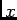position. y float The node's undistorted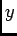position.
If flag is 1, then the node displacement is also specified:
 dx float The node'sdisplacement. dy float The node'sdisplacement.

 Linear Nodes type int The position of linear in the node name list. flag char See below. index int The node index. Must be unique, and all integers from 0 up to the number of nodes must be accounted for. x float The node's undistortedposition. y float The node's undistortedposition.
If the low bit of flag is 1, then the node displacement is also specified:
 dx float The node'sdisplacement. dy float The node'sdisplacement.
If the second bit of flag is 1 (i.e, flag=2 or flag=3, then the transformation matrix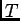is also specified.is the matrix that transforms a 2-vector in the node's coordinate system into the OOF coordinate system.
 t00 float The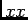component of. t01 float The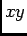component of. t10 float The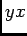component of. t11 float The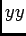component of.

The list of nodes must end with a binary integer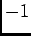:

 End List EOL int### Elements

Each element type has a different binary representation. This section of the file lists the data for each element. Because the amount of data for each type of element is known, no special termination is needed between elements.

#### Element Index

Unlike the ASCII data file, the binary data file does not contain an explicit index labeling each element. Instead, the index is inferred from the order in which the elements appear in the file. The first element is number 0, the second is 1, and so on. This index is used to specify clones and element groups.

#### Element Type

The element type is stored as an integer, indicating the position of this type in the list of element types in the header. The first element type in the list is 0, the second is 1, and so on.

#### Flag

All elements other than the empty element have an integer flag parameter. The low bit (0x1) of flag is 1 if the element is in plane strain, 0 if it's in plane stress. The second bit (0x2) is 1 if the local stiffness matrix and thermal forces are stored. The third bit (0x4) is 1 if this element is a clone (see below), and the fourth bit (0x8) is 1 if the element has been mutated.

#### Clones

The third bit (0x4) of flag is 1 if this element is a clone. To save space and time, elements can be flagged as being clones of other elements that have already been read. Clones must have identical geometries, orientations, and thermoelastic parameters. They can differ only in their node indices and gray value.6.3 The big advantage of clones is that their local stiffness matrices do not have to be stored or computed; they can simply be copied from another element. The clone index appearing in the tables below is the element index of the element whose stiffness matrix is to be copied.

In no case can both the clone index and the stiffness matrix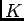be specified at the same time. The notation (conditional)'' in the tables means that the datum in question is present only if flag is set appropriately.

#### Mutated Elements

The fourth bit (0x8) of flag is 1 if the element has been mutated. The notation (mutated)'' in the tables indicates that the datum in question is present only if the element has been mutated.

If stiffness matrices or thermal forces are provided in the data file, then the data type in the file below is listed as stiffness or force. The exact form of these is listed at the end of this section.

#### Termination

The list of elements must end with a binary integer.

### Data Details for Elements

The data for each element type is as follows:

 Isotropic Element (Section 4.5.1) type int Element type. gray float Gray value used to display the element. node1 int Index of first node, going counterclockwise around the element. node2 int Index of second node. node3 int Index of third node. poisson float Poisson's ratio. young float Young's modulus. alpha float Thermal expansion coefficient. flag int See above. K stiffness Stiffness matrix (conditional). F force Thermal forces (conditional). clone int Clone index (conditional).

 empty Element (Section 4.5.2) type int Element type. gray float Gray value used to display the element. node1 int Index of first node, going counterclockwise around the element. node2 int Index of second node. node3 int Index of third node.

 cubic Element (Section 4.5.3) type int Element type. gray float Gray value used to display the element. node1 int Index of first node, going counterclockwise around the element. node2 int Index of second node. node3 int Index of third node. L float Latitude (See Section 4.3). R float Rotation. S float Spin. flag int See above. K stiffness Stiffness matrix (conditional). F force Thermal forces (conditional). clone int Clone index (conditional). poisson float Poisson's ratio. young float Young's modulus. alpha float Thermal expansion coefficient. A float Anisotropy.

 hexagonal Element (Section 4.5.4) type int Element type. gray float Gray value used to display the element. node1 int Index of first node, going counterclockwise around the element. node2 int Index of second node. node3 int Index of third node. L float Latitude (See Section 4.3). R float Rotation. S float Spin. flag int See above. K stiffness Stiffness matrix (conditional). F force Thermal forces (conditional). clone int Clone index (conditional). c11 float Elastic coefficient. c12 float Elastic coefficient. c13 float Elastic coefficient. c33 float Elastic coefficient. c44 float Elastic coefficient. alpha11 float Thermal expansion coefficient. alpha33 float Thermal expansion coefficient.

 orthorhombic Element (Section 4.5.5) type int Element type. gray float Gray value used to display the element. node1 int Index of first node, going counterclockwise around the element. node2 int Index of second node. node3 int Index of third node. L float Latitude (See Section 4.3). R float Rotation. S float Spin. flag int See above. K stiffness Stiffness matrix (conditional). F force Thermal forces (conditional). clone int Clone index (conditional). c11 float Elastic coefficient. c12 float Elastic coefficient. c13 float Elastic coefficient. c22 float Elastic coefficient. c23 float Elastic coefficient. c33 float Elastic coefficient. c55 float Elastic coefficient. c66 float Elastic coefficient. alpha11 float Thermal expansion coefficient. alpha22 float Thermal expansion coefficient. alpha33 float Thermal expansion coefficient.

 trigonalA Element (Section 4.5.7) type int Element type. gray float Gray value used to display the element. node1 int Index of first node, going counterclockwise around the element. node2 int Index of second node. node3 int Index of third node. L float Latitude (See Section 4.3). R float Rotation. S float Spin. flag int See above. K stiffness Stiffness matrix (conditional). F force Thermal forces (conditional). clone int Clone index (conditional). c11 float Elastic coefficient. c12 float Elastic coefficient. c13 float Elastic coefficient. c14 float Elastic coefficient. c15 float Elastic coefficient. c33 float Elastic coefficient. c44 float Elastic coefficient. alpha11 float Thermal expansion coefficient. alpha33 float Thermal expansion coefficient.

 trigonalB Element (Section 4.5.8) type int Element type. gray float Gray value used to display the element. node1 int Index of first node, going counterclockwise around the element. node2 int Index of second node. node3 int Index of third node. L float Latitude (See Section 4.3). R float Rotation. S float Spin. flag int See above. K stiffness Stiffness matrix (conditional). F force Thermal forces (conditional). clone int Clone index (conditional). c11 float Elastic coefficient. c12 float Elastic coefficient. c13 float Elastic coefficient. c14 float Elastic coefficient. c33 float Elastic coefficient. c44 float Elastic coefficient. alpha11 float Thermal expansion coefficient. alpha33 float Thermal expansion coefficient.

 eds_el Element (Section 4.5.6) type int Element type. gray float Gray value used to display the element. node1 int Index of first node, going counterclockwise around the element. node2 int Index of second node. node3 int Index of third node. L float Latitude (See Section 4.3). R float Rotation. S float Spin. flag int See above. K stiffness Stiffness matrix (conditional). F force Thermal forces (conditional). clone int Clone index (conditional). poisson float Poisson's ratio. young float Young's modulus. a1 float Thermal expansion coefficient. a2 float Thermal expansion coefficient. a3 float Thermal expansion coefficient.

 damisotropic Element (Section 4.5.9) type int Element type. gray float Gray value used to display the element. node1 int Index of first node, going counterclockwise around the element. node2 int Index of second node. node3 int Index of third node. poisson float Poisson's ratio. young float Young's modulus. alpha float Thermal expansion coefficient. max_s float Maximum stress. min_s float Minimum stress. max_kd float Maximum knockdown factor. min_kd float Minimum knockdown factor. flag int See above. K stiffness Stiffness matrix (conditional). F force Thermal forces (conditional). clone int Clone index (conditional). total_kd float Product of all applied knockdowns (mutated.) oldgray float Gray value before mutation (mutated).

 damage Element (Section 4.5.10) type int Element type. gray float Gray value used to display the element. node1 int Index of first node, going counterclockwise around the element. node2 int Index of second node. node3 int Index of third node. L float Latitude (See Section 4.3). R float Rotation. S float Spin. flag int See above. K stiffness Stiffness matrix (conditional). F force Thermal forces (conditional). clone int Clone index (conditional). poisson float Poisson's ratio. young float Young's modulus. alpha float Thermal expansion coefficient. max_s float Maximum stress. kd_1 float First knockdown factor. kd_2 float Second knockdown factor. only_once int Mutate only once? oldgray float Gray value before mutation (mutated). C_ijkl stiffness Mutated 3-D stiffness matrix (mutated).

 griffith and griffith2 Elements (Section 4.5.11) type int Element type. gray float Gray value used to display the element. node1 int Index of first node, going counterclockwise around the element. node2 int Index of second node. node3 int Index of third node. L float Latitude (See Section 4.3). R float Rotation. S float Spin. flag int See above. K stiffness Stiffness matrix (conditional). F force Thermal forces (conditional). clone int Clone index (conditional). poisson float Poisson's ratio of unmutated element. young float Young's modulus of unmutated element. alpha float Thermal expansion coefficient. gamma float Surface energy density. kd_1 float First knockdown factor. kd_2 float Second knockdown factor. only_once int Mutate only once? oldgray float Gray value before mutation (mutated). C_ijkl stiffness Mutated 3-D stiffness matrix (mutated).

 zimmer and zimmer2 Elements (Section 4.5.13) type int Element type. gray float Gray value used to display the element. node1 int Index of first node, going counterclockwise around the element. node2 int Index of second node. node3 int Index of third node. L float Latitude (See Section 4.3). R float Rotation. S float Spin. flag int See above. K stiffness Stiffness matrix (conditional). F force Thermal forces (conditional). clone int Clone index (conditional). c11 float Elastic coefficient. c12 float Elastic coefficient. c13 float Elastic coefficient. c33 float Elastic coefficient. c44 float Elastic coefficient. alpha11 float Thermal expansion coefficient. alpha33 float Thermal expansion coefficient. gamma float Surface energy density. kd_1 float First knockdown factor. kd_2 float Second knockdown factor. only_once int Mutate only once? oldgray float Gray value before mutation (mutated). C_ijkl stiffness Mutated 3-D stiffness matrix (mutated).

The local stiffness matrix is a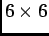symmetric matrix, giving the element's contribution to the global stiffness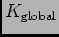. It's easiest by far to leave it out of the file. If you are writing your own binary .goof file, and really really want to include the stiffness matrices, it's best to write the file without them, load it into OOF and use /output/grid/binary (Section 3.31.2) with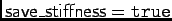to write a new .goof file. For completeness, though, here is the binary format used to save the local stiffness matrix and thermal forces:

 Stiffness Matrices k00 double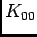: only the lower triangular part ofis stored. k10 double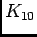k11 double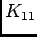k20 double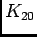k21 double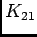k22 double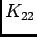k30 double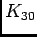k31 double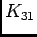k32 double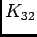k33 double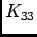k40 double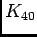k41 double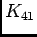k42 double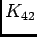k43 double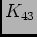k44 double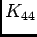k50 double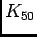k51 double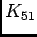k52 double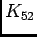k53 double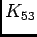k54 double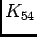k55 double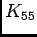Thermal Forces F0x doublecomponent of thermal force on node 0. F0y doublecomponent of thermal force on node 0. F1x doublecomponent of thermal force on node 1. F1y doublecomponent of thermal force on node 1. F2x doublecomponent of thermal force on node 2. F2y doublecomponent of thermal force on node 2.

### Node Groups

Each of the node groups is listed after the elements, in the order in which they appear in the node group name list in the header. Each list is a sequence of integers, giving the indices of the nodes in the group. Each list must end with a binary integer.

 Node Groups n1 int Index of first node in first node group. ... nn int Index of last node in first node group. EOL intn1 int Index of first node in second node group. ... etc.

### Element Groups

The element groups are written in an analogous way to the node groups. The groups must be listed in the order in which they appear in the element group name list in the header. Each list is a sequence of integers giving the element indices of the elements in the group. Each list must end with a binary integer.

 Element Groups e1 int Index of first element in first element group. ... en int Index of last element in first element group. EOL inte1 int Index of first element in second element group. ... etc.

### Startup Commands

This final section of the binary data file is again in ASCII. It is identical to the Startup Commands section of the ASCII data file: a series of arbitrary OOF commands, each preceded by the word oof.Next: Known Bugs Up: Data File Formats Previous: ASCII Format   Contents
/* Send mail to the OOF Team *//* Go to the OOF Home Page */